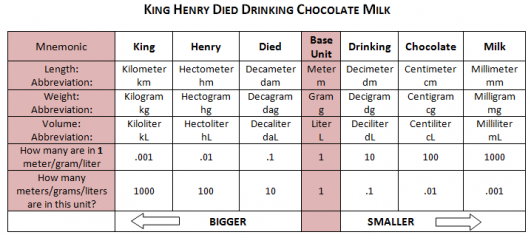# Does Dm Mean Decimeter Or Decameter?

Dm is the commonly used abbreviation for the decimeter. A decimeter is one-tenth of a meter (i.e. 10 centimeters). The decameter, sometimes referred to as the dekameter, has the abbreviation dam, or dk. A decameter is one-hundredth of a kilometer (i.e. 100 meters).The decimeter and the decameter are rarely used terms in the metric system of measurements. Mostly we get by just fine with millimeters (mm) centimeters (cm), meters (m), and kilometers (km), with even scientists and advanced mathematicians sticking to these four units, although they can lead to some difficulties, and the need for equations, when dealing with lengths of microscopic or astronomical natures.

Other Unusual Metric Terms

The megameter is the next term 'up' from the kilometer, and is equivalent to 1000 kilometers. For instance, the average distance between Earth and the Moon could be written as 384.4Mm instead of 384,400km. The micrometer, the next unit of measurement 'down' from the millimeter, is one one-thousandth of a millimeter. The micrometer has the abbreviation µm.

If can be tricky learning all the terms and abbreviations used throughout the metric systems, but there's plenty of help available online, such as this useful film:

thanked the writer.